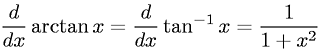Equations > Calculus > Differentiation/Differentals > Derivative of Inverse Tangent (Arctangent)

### Derivative of Inverse Tangent (Arctangent)Latex Code:

MathML Code:

 $\fracd\mathrm{dx}\mathrm{arctan}x=\fracd\mathrm{dx}{\mathrm{tan}}^{-1}x=\frac11+{x}^{2}$

MathType 5.0: